# Density of state and fermi energy in 3-D

In three-dimensional (3D) solids, the density of states describes the number of available quantum states per unit energy interval at a given energy level.

The free particle SWE in 3-D is: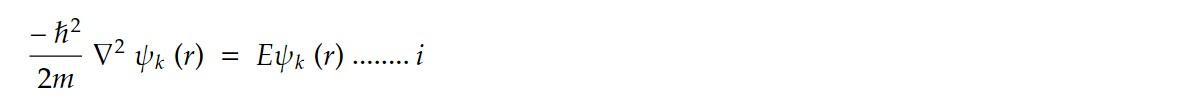Ideal crystalline solids possess translational symmetry so it is convenient to introduce wave function that satisfy the boundary condition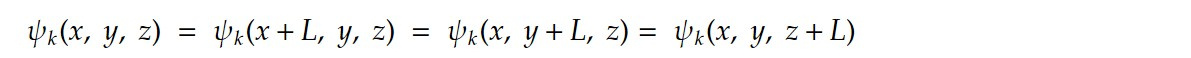The solution of equation (i) isApplying boundary condition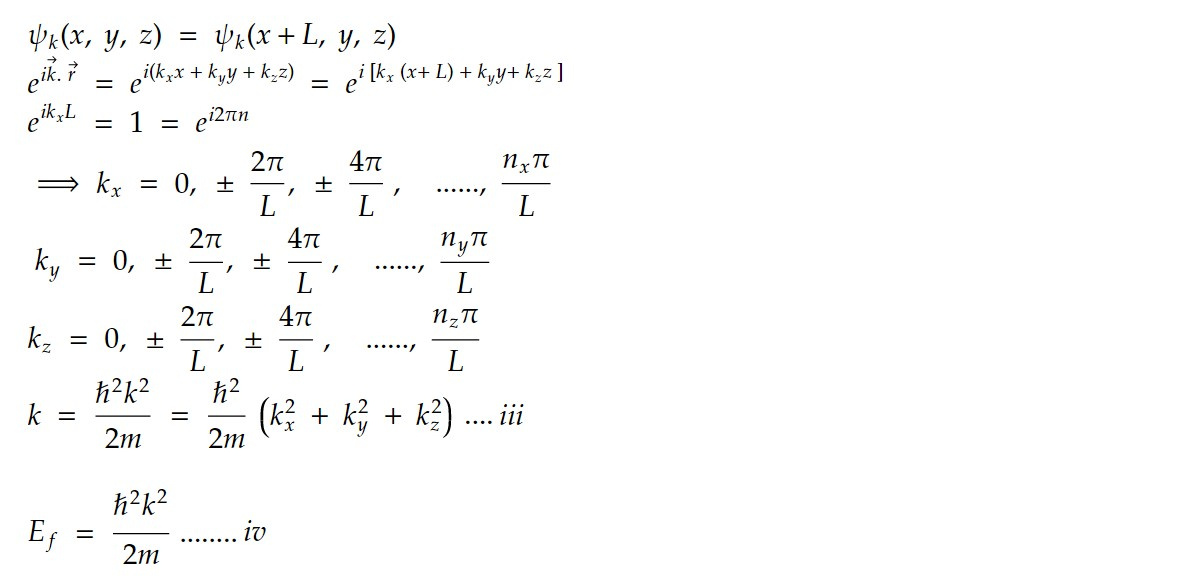In the ground state of system of N free electrons, the occupied orbitals may be represented by points inside a sphere in k space. The energy of the surface of sphere is fermi energy and wave vector at fermi surface have a magnitude kf such that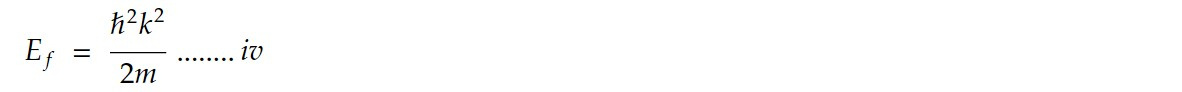The allowed values of kx, ky and kz shows that N free electrons inside the sphere in K space have total number of orbitals

N = 2 Volume of k space sphere/ Volume of element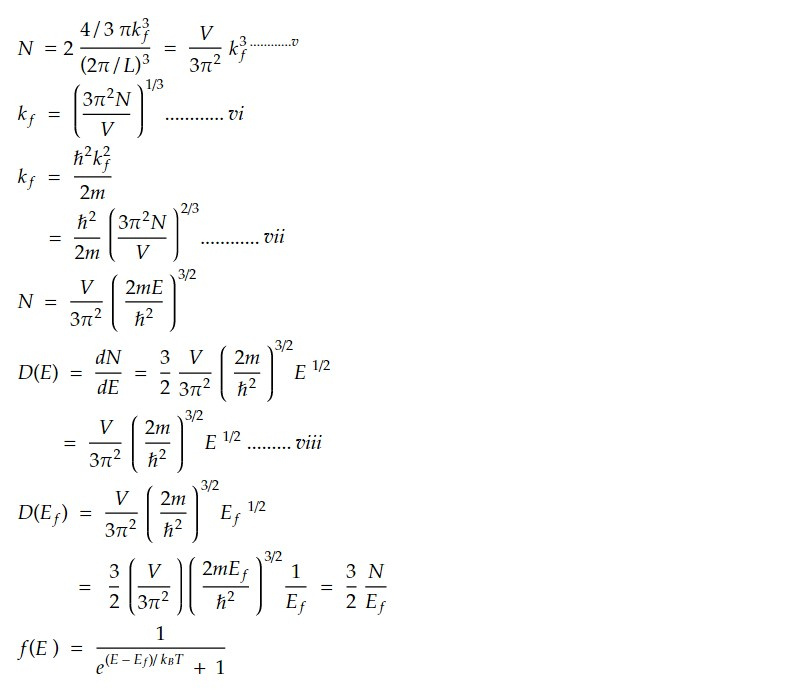Hence f(E) = 1 for T = 0k (E< Ef)

The variation of density of state with energy for free electron gas in 3 D is shown in figure. When the temperature is increased from 0 to T k, average energy increases and electron from region 1 are excited to region 2 (above Ef)

Here, the Fermi energy (Ef) is the energy level at which the probability of finding an electron is 0.5 at absolute zero temperature. At this energy, the Fermi-Dirac distribution function f(E) is equal to 0.5. The Fermi energy is an important parameter in understanding the electronic properties of solids.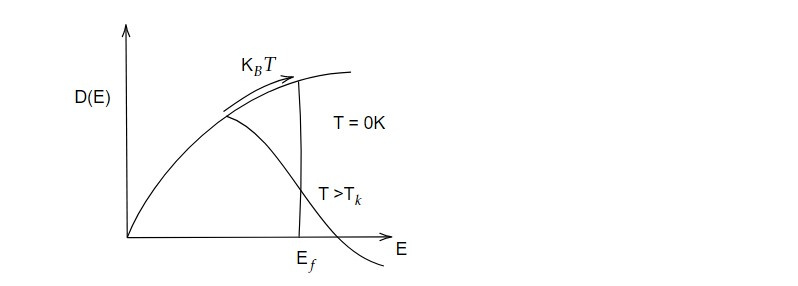The Density of state and Fermi energy are important in understanding the electrical and thermal properties of solids. For example, the electrical conductivity of a solid is related to the Density of state and Fermi energy, and the heat capacity of a solid is related to the density of states.

This note is a part of the Physics Repository.